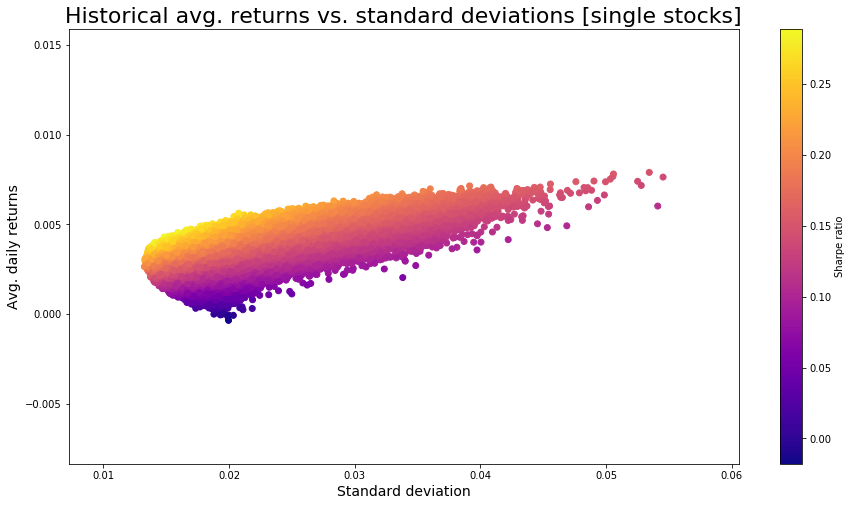# Building efficient freight & trucking stock portfolios in PythonIn previous posts I have demonstrated how you can query stock price data with e.g. pandas_datareader in Python.

In this post I will present an algorithm with which you can construct an efficient portfolio based on any set of stocks that you might be considering. The algorithm will determine the optimal share of each stock in your portfolio, based on the level of risk that you feel confident taking.

More precisely I will present a method for visualizing the efficient frontier of portfolios consisting of a specific set of stocks. In this example I will work with the following 8 stocks, which are all trucking companies:

• Yamato Holdings (YATRY)
• Knight-Swift Transportation Holdings (KNX)
• BEST (BEST)
• YRC Worldwide (YRCW)
• Schneider National (SNDR)
• Old Dominion Freight Line (ODFL)
• Arc Best (ARCB)
• Werner Enterprises (WERN)

Risk is measured as standard deviation of historical returns. Return is measured as average historical daily stock return (using closing prices).

I start by importing some relevant modules in Python:

```# import relevant modules
import pandas as pd
import numpy as np
import datetime
import matplotlib.pyplot as plt
import statistics as stat
import random as rnd
from matplotlib.ticker import StrMethodFormatter
```

I want to collect historical stock price data for the previous 6 months. Below I specify start and end date of the relevant period to collect data from:

```# specify relevant start and end date for stock price data collection period
start_date = datetime.datetime(2020,4,1)
end_date = datetime.datetime(2020,9,30)
```

Next, I define a helper function which will take in a stock price data frame collected from Yahoo Finance via pandas_datareader and translate it into daily returns:

```# define function that returns a list of daily returns
def returns(df):
prices = df["Close"]
returns = [0 if i == 0 else (prices[i]-prices[i-1])/(prices[i-1]) for i in range(0,len(prices))]
return(returns)
```

Now I define another helper function which will take in a list of stock ticks and calculate their average daily return and their standard deviation of daily returns for some specific period. This function makes use of pandas_datareader to query stock price data from Yahoo:

```# define function that can construct data frame with standard deviations and average daily returns
def analyzeStocks(tickersArr,start_date,end_date):
# create empty data frame template
index = ["ticker","return","stdev"]
muArr = []
sigmaArr = []
# loop through all tickers
for i in range(0,len(tickersArr)):
tick = tickersArr[i]
# get stock price data
# calculate average daily return
muArr.append(stat.mean(returns(data)))
# calculate standard deviation
sigmaArr.append(stat.stdev(returns(data)))
# return a data frame
return(pd.DataFrame(np.array([tickersArr, muArr, sigmaArr]),index=index,columns=tickersArr))
```

In this post I want analyze the ticks below:

```tickersArr = ["YATRY","KNX","BEST","YRCW","SNDR","ODFL","ARCB","WERN"]
```

Using above tickers I execute analyzeStocks to pull stock price data and to calulate avg. daily return and standard deviation of daily returns:

```base_df = analyzeStocks(tickersArr,start_date,end_date)
base_df
```

Using matplotlib I make a simple scatter plot of the historical return vs. volatility performance of the single stocks:

```plt.figure(figsize=(15,8))
muArr = [float(i) for i in base_df.iloc[1,]]
sigmaArr = [float(i) for i in base_df.iloc[2,]]
sharpeArr = [muArr[i]/sigmaArr[i] for i in range(0,len(muArr))]
plt.scatter(sigmaArr,muArr,c=sharpeArr,cmap="plasma")
plt.title("Historical avg. returns vs. standard deviations [single stocks]",size=22)
plt.xlabel("Standard deviation",size=14)
plt.ylabel("Avg. daily return",size=14)
```
`Text(0, 0.5, 'Avg. daily return')`

Now, I define a portfolio building function. The function will create a defined number of portfolios with randomly assigned weights per stock. The expected return and standard deviation of daily returns resulting from this are returned in the form of a pandas data frame:

```# define function for creating defined number of portfolios
def portfolioBuilder(n,tickersArr,start_date,end_date):
muArr = []
sigmaArr = []
dailyreturnsArr = []
weightedreturnsArr = []
portfoliodailyreturnsArr = []
# populate daily returns
for i in range(0,len(tickersArr)):
dailyreturnsArr.append(returns(data))
# create n different portfolios
for i in range(0,n):
# reset daily portfolio list
portfoliodailyreturnsArr = []
# create portfolio weight
weightsArr = [rnd.uniform(0,1) for i in range(0,len(tickersArr))]
nweightsArr = [i/sum(weightsArr) for i in weightsArr]
# weight the daily returns
for j in range(0,len(dailyreturnsArr)):
temp = 0
for k in range(0,len(tickersArr)):
temp = temp + float(dailyreturnsArr[k][j])*float(nweightsArr[k])
portfoliodailyreturnsArr.append(temp)
# calculate and append mavg daily weighted portfolio returns
muArr.append(stat.mean(portfoliodailyreturnsArr))
# calculate and append standard deviation of weighted portfolio's daily returns
sigmaArr.append(stat.stdev(portfoliodailyreturnsArr))
# return expected returns and standard deviation for the portfolios created
return([sigmaArr,muArr])
```

I apply the portfolio building function to the tickers and plot the outcome using for 500000 random portfolios using matplotlib.pyplot:

```portfoliosArr = portfolioBuilder(500000,tickersArr,start_date,end_date)
plt.figure(figsize=(15,8))
muArr = [float(portfoliosArr[i]) for i in range(0,len(portfoliosArr))]
sigmaArr = [float(portfoliosArr[i]) for i in range(0,len(portfoliosArr))]
sharpeArr = [muArr[i]/sigmaArr[i] for i in range(0,len(muArr))]
plt.scatter(sigmaArr,muArr,c=sharpeArr,cmap="plasma")
plt.title("Historical avg. returns vs. standard deviations [single stocks]",size=22)
plt.colorbar(label="Sharpe ratio")
plt.xlabel("Standard deviation",size=14)
plt.ylabel("Avg. daily returns",size=14)
```
`Text(0, 0.5, 'Avg. daily returns')`

This chart allows you to validate whether your current choice of weights for your stocks of choice are currently efficient or not. Efficient portfolios would be located along the upper line of the scatter plot.